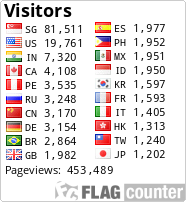# Avoid row-by-row scanning

#### Link to this posting

Don't process DataWindows and DataStores row by row if the task can be accomplished in another, more efficient way.

For example, when you are looking for a value (or values combination), use Find() function instead of row-by-row comparison.

If you want a row (for example, the current row) not to be searched (it can happen when you want to check if there are more rows with the same value(s) as in the current row), call Find() twice: from the first row to the before-skipped row, and then from the just-after-skipped to the last row.

If there can be more than one row to be found then do use a loop, but iterate using Find(), without scanning ALL rows:

*** BAD code: ***

Code: Select all
`ll_row_count = dw_emp.RowCount()for ll_row = 1 to ll_row_count       ll_curr_dept_id = dw_emp.object.dept_id[ll_row]       if ll_curr_dept_id <> al_dept_id then continue       // process the found rownext`

*** GOOD code: ***

Code: Select all
`ll_row = 0ll_row_count = dw_emp.RowCount()ls_search_expr = "dept_id=" + String(al_dept_id)do while true       ll_row = dw_emp.Find(ls_search_expr, ll_row + 1, ll_row_count)       if ll_row = 0 then exit       // process the found row       if ll_row = ll_row_count then exit // prevent eternal loop when last row satisfies search conditionloop`

Maximum value of the field in all rows

The function Describe() can be useful - for example, the following code obtains the maximum Effective Date of all rows:

Code: Select all
`ld_eff_date = Date(ids_1.Describe("Evaluate('Max(eff_date)',1)"))`

Count highlighted rows

Code: Select all
`ll_selected_count = Long(dw_1.Describe("Evaluate('Sum(if(IsSelected(), 1, 0) for all)', 1)"))`

Use a computed field instead of making the calculation in a code and assigning the results to columns in each row.

If you need to assign a same value to a column in ALL the rows (like a coefficient another column should be multiplied or divided by), make that column a computed field with a very simple expression - "1", and change that expression programmatically. Suppose, a variable ll_coef_to_divide contains the result of a calculation in PB code. Here is the bad (not efficient) solution (assuming that the field is not a computed but exists in the DW's data source):

Code: Select all
`for ll_row = 1 to ll_row_count       dw_XXX.object.coef_to_divide[ll_row] = ll_coef_to_dividenext`

To make the assignment at one stroke, the field should be a computed one. The value, returned by it, is assigned this way:

Code: Select all
`dw_XXX.object.c_coef_to_divide.Expression = String(ll_coef_to_divide)dw_XXX.GroupCalc()`Ursego
Site Admin

Posts: 125
Joined: 19 Feb 2013, 20:33

## IF you want to ((lose weight) OR (have unbelievable (brain function AND mental clarity))) THEN click: